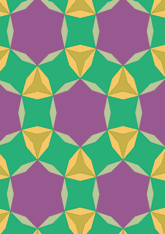# Derived from 3.4.6.4, with di-gons added, angle (180/7) degrees;data145/D346C

## Geometry

• The symmetry group of the tiling is *632 (p6m).
• All the internal angles of the constituent polygons are a multiple of 4.28 (π/42)°.
• Contains two regular two-pointed star polygons with vertex angle of 25.71 (π/7)°.
• Contains one regular three-pointed star polygon with vertex angle of 34.28 (4π/21)°.
• Contains one regular four-pointed star polygon with vertex angle of 64.28 (5π/14)°.
• Contains one regular 6-pointed star polygon with vertex angle of 94.28 (11π/21)°.
• The tiling satisfies the two-colour condition.
• The tiling is edge-to-edge.
• As drawn, contains about 183 polygons.

## References

Publications referenced:
1. Derived from figure 3.4.6.4 of B GrÃ¼nbaum and G C Shephard. Tilings and Patterns, W H Freeman, 1987. ISBN 071671193. [gands] {Most important work in this area.}
2. Uniform Star Polygons with Digons of B A Wichmann. The World of Patterns, World Scientific, 2001. ISBN 981024619. [wich2] {Only new patterns ascribed to this.}

v53